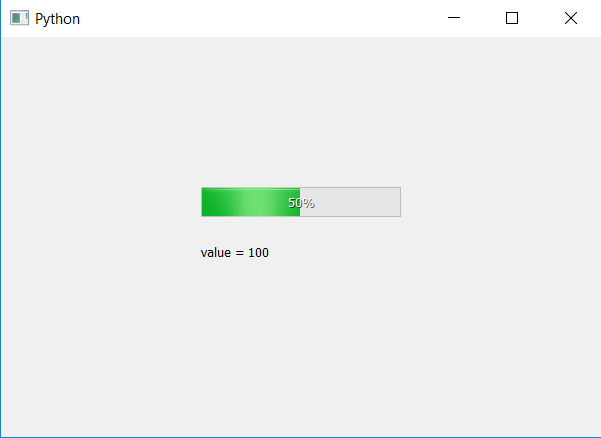# PyQt5 – How to get value of Progress Bar ?

In this article we will see how to get the value of progress bar. Value of the process bar is basically used to set the percentage of process bar.

Note : Percentage and value of process bar are both different things they can have same values but percentage depends upon the range of progress bar and value of progress bar, if range of progress bar is from 0 to 100 then percentage value will be equal to value of progress bar.

In order to get value of process bar we will use `value` method.

Syntax : bar.value()

Argument : It takes no argument.

Return : It returns integer value.

Below is the implementation.

 `# importing libraries ` `from` `PyQt5.QtWidgets ``import` `*`  `from` `PyQt5 ``import` `QtCore, QtGui ` `from` `PyQt5.QtGui ``import` `*`  `from` `PyQt5.QtCore ``import` `*`  `import` `sys ` ` `  ` `  `class` `Window(QMainWindow): ` ` `  ` `  `    ``def` `__init__(``self``): ` `        ``super``().__init__() ` ` `  `        ``# setting title ` `        ``self``.setWindowTitle(``"Python "``) ` ` `  `        ``# setting geometry ` `        ``self``.setGeometry(``100``, ``100``, ``600``, ``400``) ` ` `  `        ``# calling method ` `        ``self``.UiComponents() ` ` `  `        ``# showing all the widgets ` `        ``self``.show() ` ` `  `    ``# method for widgets ` `    ``def` `UiComponents(``self``): ` ` `  `        ``# creating progress bar ` `        ``bar ``=` `QProgressBar(``self``) ` ` `  `        ``# setting geometry to progress bar ` `        ``bar.setGeometry(``200``, ``150``, ``200``, ``30``) ` ` `  `        ``# setting maximum value of progress bar to 1000 ` `        ``bar.setMaximum(``200``) ` ` `  `        ``# setting value to progress bar ` `        ``bar.setValue(``100``) ` ` `  `        ``# getting value of progress bar ` `        ``value ``=` `bar.value() ` ` `  `        ``# creating label to print the value ` `        ``label ``=` `QLabel(``"value = "` `+` `str``(value), ``self``) ` ` `  `        ``# moving the label ` `        ``label.move(``200``, ``200``) ` ` `  `        ``# setting alignment to centre ` `        ``bar.setAlignment(Qt.AlignCenter) ` ` `  ` `  `# create pyqt5 app ` `App ``=` `QApplication(sys.argv) ` ` `  `# create the instance of our Window ` `window ``=` `Window() ` ` `  `# start the app ` `sys.exit(App.``exec``()) `

Output :Attention geek! Strengthen your foundations with the Python Programming Foundation Course and learn the basics.

To begin with, your interview preparations Enhance your Data Structures concepts with the Python DS Course.

My Personal Notes arrow_drop_upCheck out this Author's contributed articles.

If you like GeeksforGeeks and would like to contribute, you can also write an article using contribute.geeksforgeeks.org or mail your article to contribute@geeksforgeeks.org. See your article appearing on the GeeksforGeeks main page and help other Geeks.

Please Improve this article if you find anything incorrect by clicking on the "Improve Article" button below.

Article Tags :

Be the First to upvote.

Please write to us at contribute@geeksforgeeks.org to report any issue with the above content.